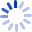Regression Diagnostics: An Introduction

by

Regression diagnostics are methods for determining whether a regression model that has been fit to data adequately represents the structure of the data. For example, if the model assumes a linear (straight-line) relationship between the response and an explanatory variable, is the assumption of linearity warranted? Regression diagnostics not only reveal deficiencies in a regression model that has been fit to data but in many instances may suggest how the model can be improved. The Second Edition of this bestselling volume ...

Cash for Textbooks